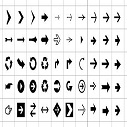# Python Iterable and Iterator

### Iterable

Iterable is something that can be looped over. For example a list.

If Something is iterable then it needs to have __iter__() method(Which is also called dunder or magic or special method).

We can check __iter__() method using dir(list)

```nums = [1, 2, 3]

for num in nums:
print(num)

print(dir(nums))
```

In above example, in background, for loop is calling __iter__() method on an object(list) and returning an iterator that we can loop over.

### Iterator

An iterator is an object with a state so that it remembers where it is, during iteration, it also knows how to get next value using __next__() method.

A list is iterable but its not an iterator, but if we run dunder iter method(__iter__()) on a list, it returns an iterator.

```nums = [1, 2, 3]

print(next(nums))  # It will give error as its not an iterator

# Make it iterator

i_nums = iter(nums)  # iter function calls nums.__iter__() in background
print(i_nums)  # Now its an iterator
print(dir(i_nums))  # Now this has __next__() method in it as it is an iterator
```

Iterators are also iterables but the difference is __iter__() method returns the same object for iterator.

As iterators remember the state, it will print next value after each time we call next(). It throws StopIteration Exception when it runs out of values.

```for num in i_nums:
print(num)
1
2
3

# Or

print(next(i_nums)) # 1
print(next(i_nums)) # 2

```

In background the for loop doing Something like this

```while True:
try:
item = next(i_nums)
print(item)
except StopIteration:
break
```

### Let's crreate a class that behaves like builtin range() function.

```class MyRange:
def __init__(self, start, end):
self.value = start
self.end = end

def __iter__(self):  # For something to be iterable, it needs to have __iter__() method
return self

def __next__(self):  # For something to be iterator, it needs to have __next__() method
if self.value >= self.end:
raise StopIteration
current = self.value
self.value += 1
return current

nums = MyRange(1, 10)
for num in nums:
print(num)

# Or

print(next(nums))   # next() method calls __next__() in background
print(m.__next__())
print(m.__next__())
print(m.__next__())
```

### Genrators

Generators are also iterators but __iter__ and __next__ methods are created automatically for them.

```def my_range(start, end):
current = start
while current < end:
yield current
current += 1

nums = my_range(1, 10)

for num in nums:
print(num)

# Or
print(next(nums))
print(next(nums))
print(next(nums))
print(next(nums))
```

### Creating our own iterators, iterable

```class Sentence:

def __init__(self, sentence):
self.words = sentence.split()
self.index = 0

def __iter__(self):
return self

def __next__(self):
print(f'Index is {self.index}')
if self.index >= len(self.words):
raise StopIteration
current = self.index
self.index += 1
return self.words[current]

my_sentance = Sentence('This is a test')
for word in my_sentance:
print(word)

# Or

print(next(my_sentance))
print(next(my_sentance))
print(next(my_sentance))
print(next(my_sentance))
```

#### Now create generator which does the same this

```def sentence(words):
for word in words.split():
yield word

my_sentance = sentence('This is a test')
for word in my_sentance:
print(word)

# Or

print(next(my_sentance))
print(next(my_sentance))
print(next(my_sentance))
print(next(my_sentance))

```Nupur on Sep 27, 2020 at 06:09 am

This article is contributed by Nupur. If you like dEexams.com and would like to contribute, you can write your article here or mail your article to admin@deexams.com . See your article appearing on the dEexams.com main page and help others to learn.

#### Comments( 0)

##### WEB TECHNOLOGY##### TypeScript Programmning Language##### New HTML5 APIs##### HTML Arrow -> Range: Decimal 8592-8703. Hex 2190-21FF.##### How To Override One CSS Class With Another×

## Forgot Password

Please enter your email address below and we will send you information to change your password.